# Expected Shortfall

## Definition

Expected Shortfall (ES) is a Risk Measure used in the context of Quantitative Risk Management of Market Risk or Credit Risk in a Portfolio Management context. The "expected shortfall at level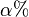$\alpha %$" is the Expected Loss experienced in the portfolio in the worst$\alpha \%$ of scenarios.

ES is an alternative to Value at Risk that is addressing some widely criticized attributes of VaR as a risk measure. Expected shortfall is also called conditional value at risk (CVaR), average value at risk (AVaR), and expected tail loss (ETL).

## Formula

If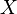$X$ is a Random Variable expressing the Risk Distribution of a portfolio at some future time and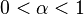$0 < \alpha < 1$ then we define the expected shortfall as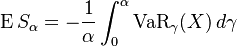$\operatorname ES_\alpha = -\frac{1}{\alpha} \int_0^\alpha \operatorname{VaR}_\gamma(X) \, d\gamma$

where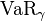$\operatorname{VaR}_\gamma$ is the Value at Risk.